# Modeling a System

Publish Date: Oct 10, 2012 | 9 Ratings | 4.56 out of 5 | Print | Submit your review

## Overview

LabVIEW can be used to represent a physical system or a model. This tutorial shows how to enter a differential equation model into LabVIEW using the LabVIEW Control Design and Simulation Module.

These tutorials are based on the Control Tutorials developed by Professor Dawn Tilbury of the Mechanical Engineering department at the University of Michigan and Professor Bill Messner of the Department of Mechanical Engineering at Carnegie Mellon University and were developed with their permission.

## Table of Contents

### 1. Train System

In this example, we will consider a toy train consisting of an engine and a car. Assuming that the train only travels in one direction, we want to apply control to the train so that it has a smooth start-up and stop, along with a constant-speed ride.

The mass of the engine and the car will be represented by M1 and M2, respectively. The two are held together by a spring, which has the stiffness coefficient of k. F represents the force applied by the engine, and the Greek letter, mu (which will also be represented by the letter u), represents the coefficient of rolling friction.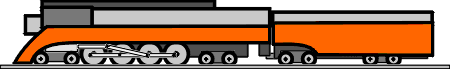### 2. Free Body Diagram and Newton's Law

The system can be represented by following Free Body Diagrams.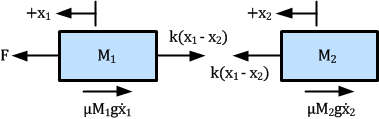Figure 1: Free Body Diagrams

From Newton's law, you know that the sum of forces acting on a mass equals the mass times its acceleration. In this case, the forces acting on M1 are the spring, the friction and the force applied by the engine. The forces acting on M2 are the spring and the friction. In the vertical direction, the gravitational force is canceled by the normal force applied by the ground, so that there will be no acceleration in the vertical direction. The equations of motion in the horizontal direction are the following: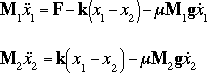### 3. State-variable and Output Equations

This set of system equations can now be manipulated into state-variable form. The state variables are the positions, X1 and X2, and the velocities, V1 and V2; the input is F. The state variable equations will look like the following: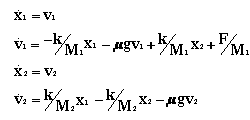Let the output of the system be the velocity of the engine. Then the output equation will be: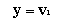### 4. Transfer Function

To find the transfer function of the system, we first take the Laplace transforms of the differential equations.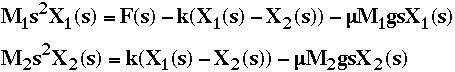The output is Y(s) = V1(s) = s X1(s). The variable X1 should be algebraically eliminated to leave an expression for Y(s)/F(s). When finding the transfer function, zero initial conditions must be assumed. The transfer function should look like the one shown below.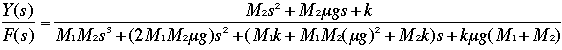### LabVIEW Graphical Approach

If you choose to use the transfer function, create a blank VI and add the CD Construct Transfer Function Model VI to your block diagram. This VI is located in the Model Construction section of the Control Design palette.

Click the drop-down box that shows “SISO” and select “Single-Input Single-Output (Symbolic)”. To create inputs for this transfer function, right-click on the Symbolic Numerator terminal and select Create » Control. Repeat this for the Symbolic Denominator and Variables terminals. These controls will now appear on the front panel.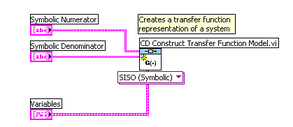Figure 2: Create Transfer Function

Next, add the CD Draw Transfer Function VI to your block diagram, located in the Model Construction section of the Control Design palette. Connect the Transfer Function Model output from the CD Create Transfer Function Model VI to the Transfer Function Model input on the CD Draw Transfer Function VI.

Finally, create an indicator from the CD Draw Transfer Function VI. To do this, right-click on the Equation terminal and select Create»Indicator.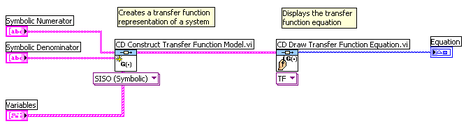Figure 3: Display Transfer Function

Now create a While Loop, located in the Structures palette, and surround all of the code in the block diagram. Next, right-click on the Loop Condition terminal in the bottom-right corner of the While Loop, and select Create»Control.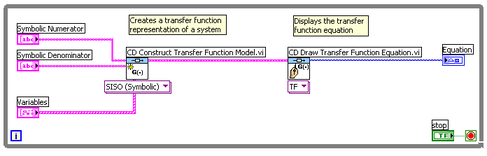Figure 4: Transfer Function with While Loop

With this VI, you can now create a transfer function for the train system. Try changing the numerator and the denominator in the front panel, and observe the effects on the transfer function equation.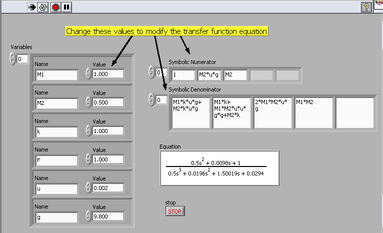Figure 5: Transfer Function Front Panel (Download)

### 5. State-Space Model

Another method to solve the problem is to use the state-space form. Four matrices A, B, C, and D characterize the system behavior and will be used to solve the problem. The state-space form which is found from the state-variable and the output equations is shown below.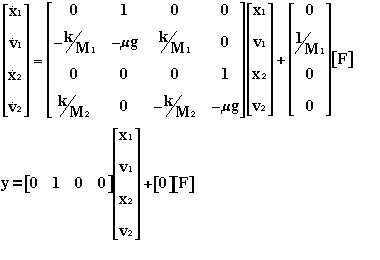### LabVIEW Graphical Approach

To model the system using the state-space form of the equations, use the CD Construct State-Space Model VI with the CD Draw State-Space Equation VI.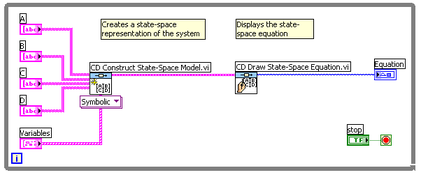Figure 6: State-Space Model Block Diagram

With this VI, you can now create a state-space model for the train system. Try changing the terms in the front panel, and observe the effects on the state-space model.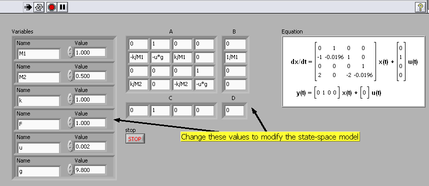Figure 7: State-Space Model Front Panel (Download)

### Hybrid Graphical/MathScript Approach

Alternatively, you can use a MathScript Node to create the state-space model. To do this, create a blank VI and insert a MathScript Node from the Structures palette. Copy and paste the following m-file code into the MathScript Node:

A=[ 0 1 0 0;

-k/M1 -u*g k/M1 0; 0 0 0 1;

k/M2 0 -k/M2 -u*g];

B=[ 0; 1/M1; 0; 0];

C=[0 1 0 0];

D=;

sys= ss(A,B,C,D);

Next, right-click on the left border of the MathScript Node and select “Add Input”. Name the input “M1”. Repeat this process to create inputs for M2, k, u, and g.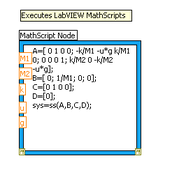Figure 8: MathScript Node

Right-click on the right border of the MathScript Node and select “Add Output” to create an output called “sys”. After creating this output, right-click on it and select Choose Data Type » Add-ons » SS object.

Next, right-click on each input and select Create » Control.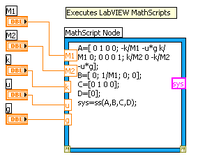Figure 9: MathScript Node with Inputs

Add the CD Draw State-Space Equation VI to the block diagram, and create an equation indicator. Connect the “sys” output from the MathScript Node to the “State-Space Model” input of the CD Draw State-Space Equation VI.

Finally, create a While Loop around the code, and create a control for the Loop Condition terminal.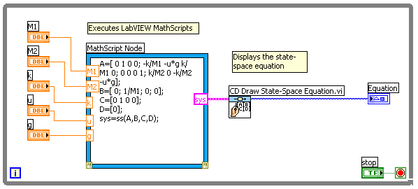Figure 10: Using MathScript Node to Create State-Space Equation

With this VI, you can now create a state-space model for the train system. Try changing the terms in the front panel, and observe the effects on the state-space model.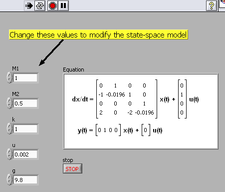Figure 11: State-Space Equation Front Panel (Download)

### Continue Solving the Problem

Once the differential equation representing the system model has been created in LabVIEW in the transfer function or state-space form, the open-loop and closed-loop system behavior can be studied.

### Ratings

Rate this document

Answered Your Question?
Yes No